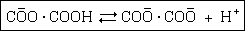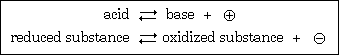# Some Remarks on the Concept of Acids and Bases

by J. N. Brønsted Recueil des Travaux Chimiques des Pays-Bas (1923), Volume 42, Pages 718-728

Toward a definition of acids and bases. Since the establishment of the theory of electrolytic dissociation by Arrhenius the dominant definition of acids and bases as substances that can split out hydrogen or hydroxyl ions in solution has never been seriously attacked so far as the definition of acids is concerned. We have held steadily to the idea that compound A is an acid if it is partly or completely broken down in solution according to the scheme

 A ---> B + H+ (1)
However, there have been attempts from various sides to modify our concept of bases. P. Pfeiffer, especially, on the basis of his important observations on aquo and hydroxo compounds in a series of metals, has presented the view that bases form salts by addition of acids, which in terms of the theory of electrolytic dissociation must lead to the idea of a definition of bases as substances that can add hydrogen ions. Although this method of treatment can be carried out convincingly here, yet in spite of its constitutive foundation it cannot serve as a direct foundation for changing the definition of a base.
The question has been treated more generally by Michaelis in his book Hydrogen Ion Concentration. He considers the following to be a possible definition of a base: a base is a special electrically neutral species of molecule that can bind a hydrogen ion and thus become a positive ion. However, the further developments by Michaelis are all founded on the usual definition of a base and particularly emphasize the special significance of hydroxyl ion as a constituent of bases even in nonaqueous solutions.
It is the purpose of the present small contribution to show the advantages that come from a modified definition of a base.
The formal advantages of the definition of a base as a substance that can bind a hydrogen ion are immediately apparent. In scheme (1) in which the concept of an acid (A) is established, the concept of a base (B) is equally defined and thus the reciprocal connection of acidic and basic properties is shown in the clearest and simplest way.
The advantages of scheme (1) as a basis for an equal definition of bases and acids are not merely of a formal nature, however. For example, if we express the basic properties of ammonia in one case by equation (1) [this appears to be a misprint; it should be 2]
 NH4+ <===> NH3 + H+ (2)
and in another by the usual formulation
 NH4+ + OH¯ <===> NH4OH (3)
then, as is well known, both of these schemes are thermodynamically equivalent for an aqueous solution, i.e., we can derive identical equilibrium conditions, from the thermodynamic viewpoint, from the basic and acidic properties that appear in dilution, partial neutralization, etc. by using (2) and (3). The presence of the compound NH4OH, that is, hydrated ammonia, and therefore the formation according to (3) of the hydroxyl ion in an ammonia solution is naturally limited to solutions in water.
This brings out the fundamental difference of the basic function as shown by (2) or (3). If we accept scheme (3),as a suitable expression for characterizing bases, we will be forced to give a special definition of a base for each special solvent. However, in principle, acid and basic properties are independent of the nature of the solvent, and the concepts of acids and bases are in fact of such a general character that we must consider it a necessary requirement of these concepts in general to formulate a pattern independent of the nature of an arbitrary solvent. Therefore we will exclude scheme (3). Scheme (2) remains for the basic property of ammonia, and for the basic function in general scheme (1) is the single foundation for an absolute definition of a base.
The result of these considerations can be made more precise in the following way: acids and bases are substances that are capable of splitting off or taking up hydrogen ions, respectively. The essence of this concept of defining acids and bases is once more given schematically by the expression
 A <===> B + H+ (1) (acid) (base)
in which nothing more is said of the properties of A and B. We will refer to A and B, that is, to an acid and a base that are related to each other by the relation given in (1), as corresponding acids and bases.
It follows from the form of the expression that corresponding acids and bases do not have to have the same state of electrical charge. If A is electrically neutral, as an ordinary acid molecule is, B has a negative charge, e.g.
 CH3COOH <===> CH3COO¯ + H+ (acid) (base)
where the acetate ion thus functions as a base. If A has a positive charge, B must be electrically neutral as is the case, e.g., of the dissociation of the ammonium ion:
 NH4+ <===> NH3 + H+ (acid) (base)
We have a negatively charged acid, e.g., in the acid oxalate ion which dissociates as follows:etc.
It also follows from the concept of a general definition of acids and bases that ions also have to be treated as acids and bases. In principle, the electrical nature of the acid or base molecule in this view has no meaning. It would be useful from a practical point of view to be able to classify the different equilibria according to charge, and I have therefore introduced the following terminology [Table I] for this purpose:
 Table I Electrical charge Naming of acidor base Examples Positive cation acid NH4+ Positive cation base NH2 . C6H4 . C6H4 . NH3+ Neutral neutral acid CH3COOH Neutral neutral base NH3 Negative anion acid COO . COOH [Note added:there is a bar abovethe second O from the leftas in the graphic above] Negative anion base CH3COO¯
Independent of the special electrical nature of the acid-base system, scheme (1) shows that the symmetry that we have seen as essential for acid and base function in the usual representation disappears in the new method of representation. However, it becomes expressed directly through the definite example of the reciprocal behavior of acids and bases.
The equilibrium formulated in scheme (1) between hydrogen ion and the corresponding acid and base can be called a simple acid-base equilibrium. By mixing two simple systems, a double acid-base system and an acid-base equilibrium result that can always be formulated as follows:
 acid1 + base2 <===> acid2 + base1 (4)
This equilibrium includes a number of important reactions such as neutralization, hydrolysis, indicator reactions, etc.
[The author then shows the specific application of his theory in a number of cases. He concludes with the summary.]
Summary. The chemical character of acids and bases is most simply and generally defined by the scheme
acid <===> base + H+
The hydroxyl ion in principle has no special position as a bearer of basic properties.
We have no logical measure for a comparison of the strengths of acids with those of bases. The usual concept of neutrality does not exist, or only by arbitrary definition. Therefore the solvent, e.g., water, cannot be considered as neutral in principle.
The acid-base and reduction-oxidation equilibria are analogous:In the first case the positive, in the second the negative electron is involved.

source: http://www.chemteam.info/Chem-History/Bronsted-Article.html